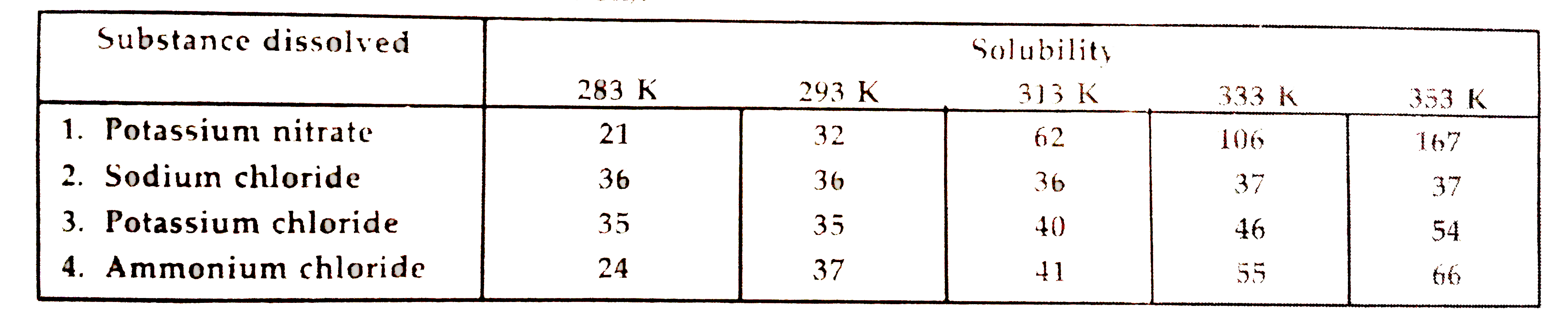# Pargya tested the solubility of four different substances at different temperatures and collected the data as given below (results are given in the fo

69 views

closed
Pargya tested the solubility of four different substances at different temperatures and collected the data as given below (results are given in the following table as grams of substance dissolved in 100 grams of water to from a saturated solutin).(a) What mass of potassium nitrate would be needed to procuce a strurated solution of potassium nitrate in 50 grams of water at 313 K ?
(b) Pragya makes a saturated solution of potassium chloride in water at 353 K and leaves the solution to cool at room temperature. What would she observe as the solution cools ? Explain.
(c ) Find the solubility of each salt at 293 K. Which salt has the highest solubility at this twmperature?
(d) What is the effect of change in temperature on the solubility of a salt ?

by (49.8k points)
selected by

(a) The solubility of potassium nitrate at 313 K is 62 grams (see the given table). This means that 62 grams of potassium nitrate is needed to make a saturated solution of potassium nitrate in 100 grams of water at 313 K. So, to make a saturated solution in 50 grams of water we eill need half of 62 grams of potassium nitrate, which is (62)/(2)=31 grams of potassium nitrate.
(b) When a saturated solution of potassium chloride at 353 K is left to cool, then solid potassium chloride (or crystals of potassium chloride) will gradually separate out from thr solution (because the solubility decreases on cooling).
(c )The solubility of various salts at 293 K is: Potassium nitrate 32 g, Sodium chloride 36 g, Potassium chloride 35 g, and Ammonium chloride 37 g. Ammonium chloride has the highest solubility (of 37 g) at this temperature of 293 K.
(d) The given data shows that the solubility of a salt increases on increasing the temperature.